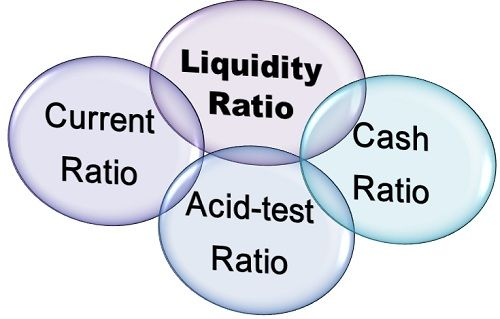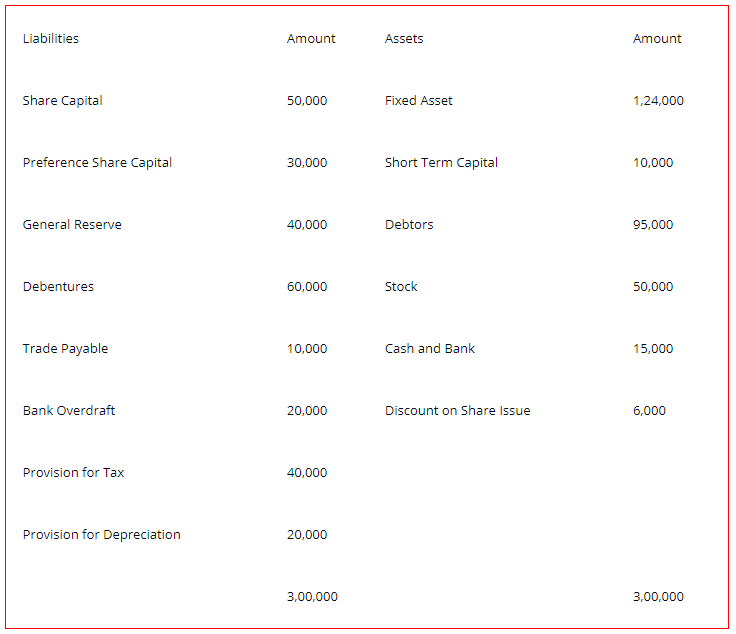# Liquidity Ratios: Current, Quick & Absolute Cash Ratio, Solved Examples

The compilation of these Accounting Ratios Notes makes students exam preparation simpler and organised.

## Liquidity Ratios

Accounting ratios are important because they assist the management in their day-to-day financial decisions. They also help them evaluate the performance of the firm and make any changes that are deemed necessary. One aspect that the management has to focus on is to ensure that the firm maintains a certain level of liquidity. Liquidity ratios help them determine that. Let us study them.

### Liquidity Ratios

A firm has assets and liabilities to its name. Some are fixed in nature and then there are current assets and current liabilities. These are short-term in nature and easily convertible into cash. The liquidity ratios deal with the relationship between such current assets and current liabilities.

Liquidity ratios evaluate the firm’s ability to pay its short-term liabilities, i.e. current liabilities. It shows the liquidity levels, i.e. how many of their assets can be quickly converted to cash to pay of their obligations when they become due.

It is not only a measure of how much cash there is but also how easily current assets can be converted to cash or marketable securities. Now let us look at some of the important liquidity ratios.Current Ratio
The current ratio is also known as the working capital ratio. It will measure the relationship between current assets and current liabilities. It measures the firm’s ability to pay for all its current liabilities, due within the next one year by selling off all its current assets. The formula is as follows

Current Ratio = CurrentAssets/CurrentLiabilities

Current Assets include

• Stock
• Debtors
• Cash and Bank Balances
• Bills receivable
• Accruals
• Short term loans that are given
• Short term Securities

Current Liabilities include

• Creditors
• Outstanding Expenses
• Short Term Loans that are taken
• Bank Overdrafts
• Provision for taxation
• Proposed Dividend

The ideal current ratio, according to the industry standard is 2:1. That means that a firm should hold at least twice the number of current assets than it has current liabilities. However, if the ratio is very high it may indicate that certain current assets are lying idle and not being utilized properly. So maintaining the correct balance between the two is crucial.

Quick Ratio
The other important one of the liquidity ratios is the Quick Ratio, also known as a liquid ratio or acid test ratio. This ratio will measure a firm’s ability to pay off its current liabilities (minus a few) by only selling off its quick assets.

Now Quick assets are those which can be easily converted to cash with only 90 days’ notice. Not all current assets are quick assets. Quick assets generally include cash, cash equivalents, and marketable securities. The formula is

Quick Ratio = $$\frac{\text { Quick Assets }}{\text { CurrentLiabilities } / \text { QuickLiabilities }}$$
Quick Assets = All Current Assets – Stock – Prepaid Expenses
Quick Liabilities = All Current Liabilities – Bank Overdraft – Cash Credit

The ideal quick ratio is considered to be 1:1, so that the firm is able to pay off all quick assets with no liquidity problems, i.e. without selling fixed assets or investments. Since it does not take into consideration stock (which is one of the biggest current assets for most firms) it is a stringent test of liquidity. Many firms believe it is a better test of liquidity than the current ratio since it is more practical.

Absolute Cash Ratio
This is an even more rigorous liquidity ratio than the quick ratio. Here we measure the availability of cash and cash equivalents to meet the short-term commitment of the firm. We do not consider all current assets, only cash. Let us see the formula,

Absolute Cash ratio = $$\frac{\text { Cash }+\text { BankBalance }+\text { MarketableSecurities }}{\text { CurrentLiabilities }}$$

As you can see, this ratio measures the cash availability of the firm to meet the current liabilities. There is no ideal ratio, it helps the management understand the level of cash availability of the firm and make any changes required.

However, if the ratio is greater than 1 it indicates poor resource management and very high liquidity. And high liquidity may mean low profitability.

Examples:

Question 1.
Given Below is the Balance sheet of ABC Co. Analyze the Balance Sheet and Calculate the Current Ratio.Solution:
Current Ratio = $$\frac{\text { CurrentAssets }}{\text { CurrentLiabilities }}$$
Current Assets = Debtors + Stock + Cash + Short term Capital = 1,70,000
Current Assets = Trade Payables + Bank Overdraft + Provision for Taxes + Provision for Depreciation = 90,000
Current Ratio = $$\frac{170000}{90000}$$ = 1.889 : 1

Question 2.
Calculate Liquid Ratio from the given details.Solution:
Quick Ratio = $$\frac{\text { QuickAssets }}{\text { CurrentLiabilities } / \text { QuickLiabilities }}$$
Quick Assets = All Current Assets – Stock – Prepaid Expenses
= 85000 – (20000 + 5000 + 10000)
= 50,000
Quick Liabilities = All Current Liabilities – Bank Overdraft – Cash Credit = 65,000
Quick Ratio = $$\frac{50000}{65000}$$ = 0.77 : 1